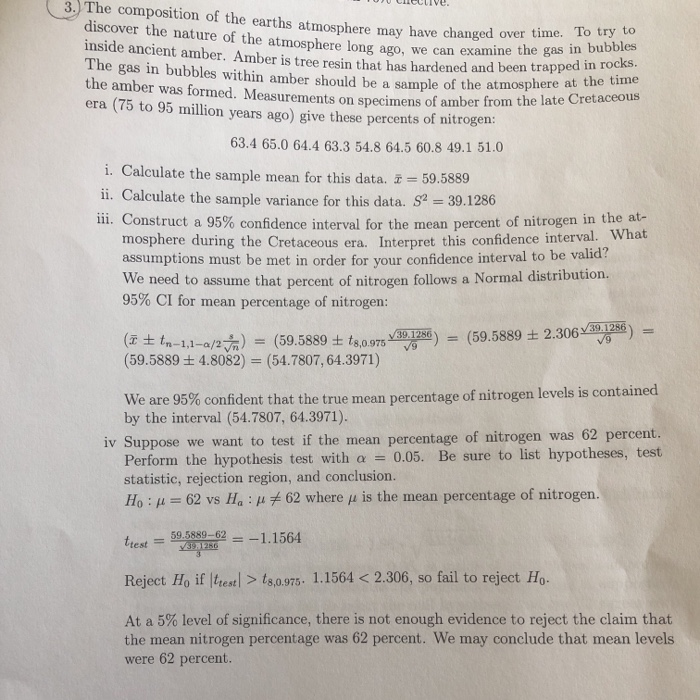# Solved: 3. The Composition Of The Earths Atmosphere May Have Changed Discover The Nature Of The Atmosphere Long Ago, We Can Examine The Gas In Bubbles Inside Ancient Amber. Amber Is Tree Resin That Ha

By |3. The composition of the earths atmosphere may have changed discover the nature of the atmosphere long ago, we can examine the gas in bubbles inside ancient amber. Amber is tree resin that has hardened and been trapped in rocks. The gas in bubbles within amber should be a sample of the atmosphere at the time the amber was formed. Measurements on specimens of amber from the late Cretaceous (75 to 95 million years ago) give these percents of nitrogen: over time. To try to era 63.4 65.0 64.4 63.3 54.8 64.5 60.8 49.1 51.0 i. Calculate the sample mean for this data. 59.5889 ii. Calculate the sample variance for this data. S2=39.1286 11i. Construct a 95% confidence interval for the mean percent of nitrogen in the at- mosphere during the Cretaceous era. Interpret this confidence interval. What assumptions must be met in order for your confidence interval to be valid? We need to assume that percent of nitrogen follows a Normal distribution. 95% CI for mean percentage of nitrogen: (59.5889 2.30639.1286 V9 V V39.1286. (t tn-1,1-a/2m (59.5889 4.8082) (54.7807, 64.3971) (59.5889 t ts,0.975 percentage of nitrogen levels is contained We are 95% confident that the true mean by the interval (54.7807, 64.3971). we want to test if the mean percentage of nitrogen was 62 percent. 0.05. Be sure to list hypotheses, test iv Suppose Perform the hypothesis test with a statistic, rejection region, and conclusion. Ho u62 vs percentage of nitrogen. 62 where u is the mean Ha: 59.5889-62 V39.1286 -1.1564 trest Reject Ho if test> ts,0.975. 1.1564 < 2.306, so fail to reject Ho At a 5% level of significance, there is not enough evidence to reject the claim that the mean nitrogen percentage was 62 percent. We may conclude that mean levels were 62 percent.